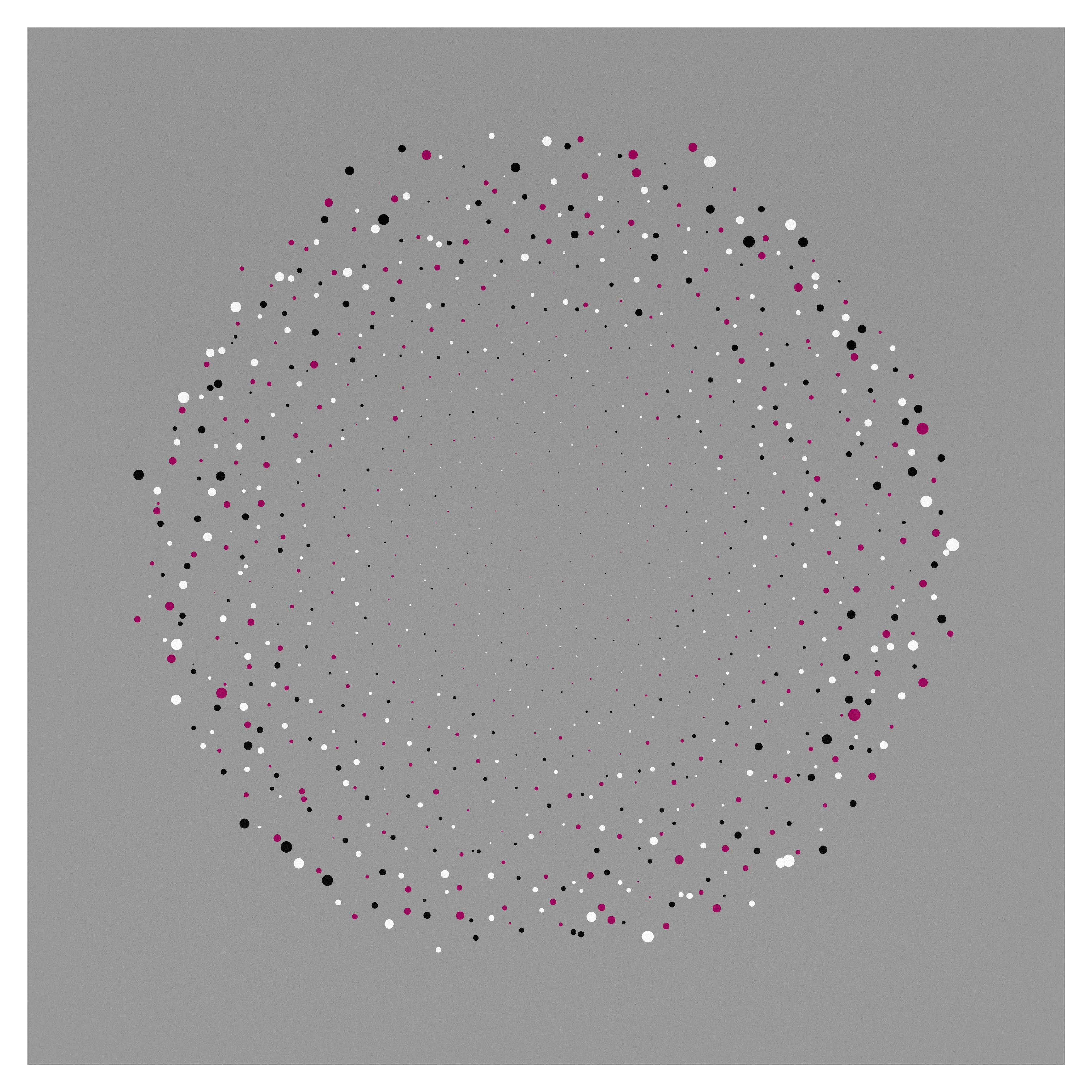I made this piece as part of the “Concentrated Creativity” BASB alumni sprint.

It is a piece of generative art made with the Clojure programming language and the Quil and genartlib libraries. The base code is inspired by the Golden Ratio Flower by Jack Rusher.

The part of my code that actually draws the above is:

``````(def palette (cycle [[326   100 61]
[0     0   0]
[0     0   100]]))

(def PHI (/ (+ 1 (Math/sqrt 5)) 2))

(defn gauss
[mean variance]
(+ mean (* variance (q/random-gaussian))))

(defn actual-draw []
; art goes here
(q/frame-rate 10)
(q/no-stroke)
(q/background 0 0 60)
(q/with-translation [(w 0.5) (h 0.5)]
(doseq [i (range 1000)]
(let [v   (+ (mod (q/frame-count) 3) i)
ang (* v PHI q/TWO-PI)
r   (* (Math/sqrt v) (w) (/ (gauss 85 2.5)))
x   (* (q/cos ang) r)
y   (* (q/sin ang) r)
sz  (+ 3 (* i (gauss 0.02 0.01)))]
(apply q/fill (nth palette i))
(q/ellipse x y sz sz))))
(doseq [x (range 0 (w) 1)
y (range 0 (h) 1)
:let [value (a/rescale (gauss y (+ y (h 0.1))) 0 (h) 0 100)]]
(q/fill 0 0 value 0.075)
(q/ellipse (gauss x (w 0.1)) (gauss y (h 0.1)) 1 1))
(q/stroke 0 0 100)
(q/stroke-weight (w 0.05))
(q/no-fill)
(q/rect 0 0 (w) (h)))
``````

I’ll work on getting the project repo on GitHub soon.